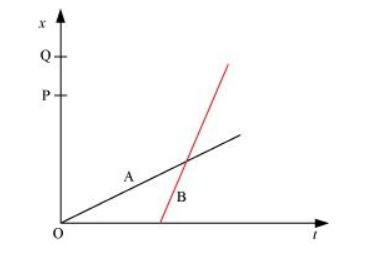# The position-time $(x-t)$ graphs for two children $A$ and $B$

Question.
The position-time $(x-t)$ graphs for two children $A$ and $B$ returning from their school $O$ to their homes $P$ and $Q$ respectively are shown in Fig.

3.19. Choose the correct entries in the brackets below;

(a) (A/B) lives closer to the school than (B/A)

(b) (A/B) starts from the school earlier than (B/A)

(c) (A/B) walks faster than (B/A)

(d) A and B reach home at the (same/different) time

(e) (A/B) overtakes (B/A) on the road (once/twice).solution:
(a) A lives closer to school than B.

(b) A starts from school earlier than B.

(c) B walks faster than A.

(d) A and B reach home at the same time.

(e) B overtakes A once on the road.

(a) In the given $x-t$ graph, it can be observed that distance $\mathrm{OP}<\mathrm{OQ}$. Hence, the distance of school from the A's home is less than that from B's home.

(b) In the given graph, it can be observed that for $x=0, t=0$ for $\mathbf{A}$, whereas for $x=0, t$ has some finite value for $\mathbf{B}$. Thus, $\mathbf{A}$ starts his journey from school earlier than $\mathbf{B}$.

(c) In the given $x-t$ graph, it can be observed that the slope of $B$ is greater than that of $A$. Since the slope of the $x-t$ graph gives the speed, a greater slope means that the speed of $\mathbf{B}$ is greater than the speed $\mathbf{A}$

(d) It is clear from the given graph that both $\mathbf{A}$ and $\mathbf{B}$ reach their respective homes at the same time.

(e) B moves later than A and his/her speed is greater than that of A. From the graph, it is clear that B overtakes A only once on the road.